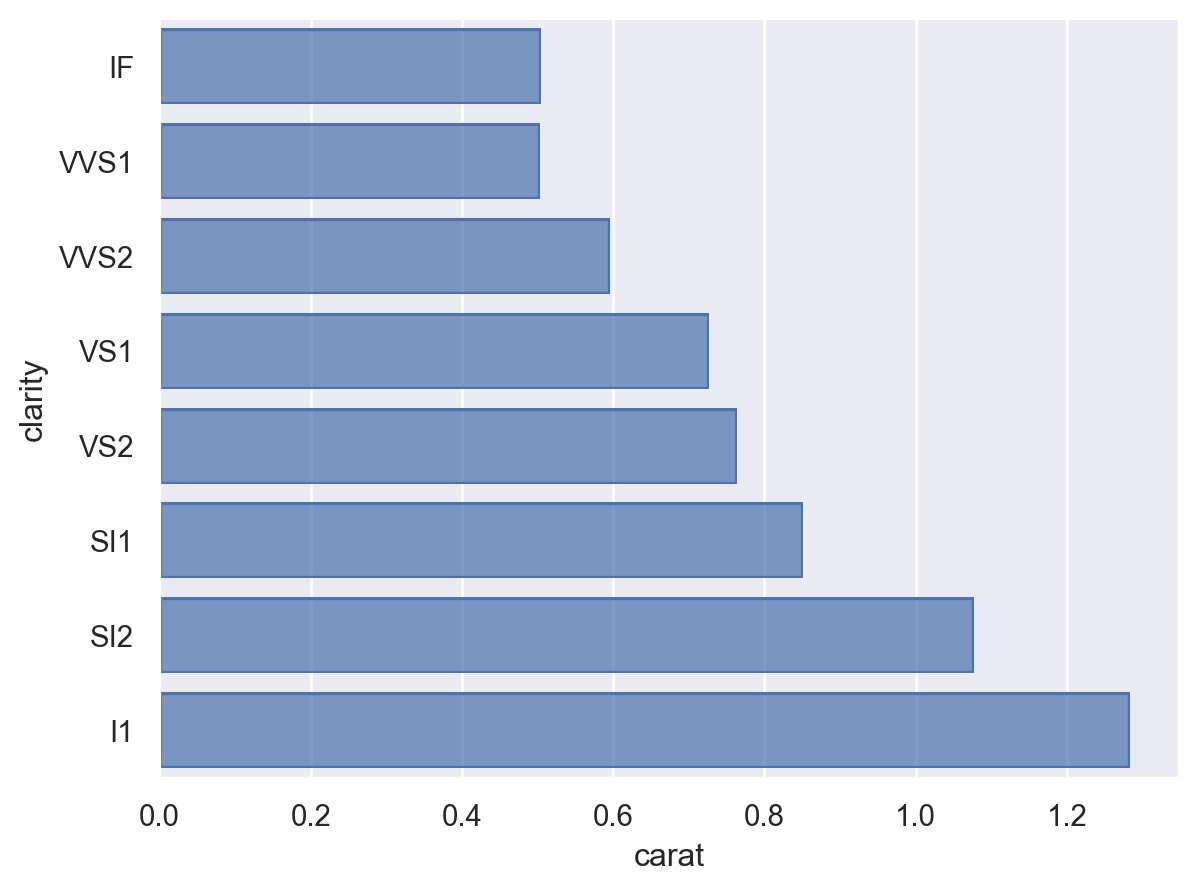# seaborn.objects.Agg#

class seaborn.objects.Agg(func='mean')#

Aggregate data along the value axis using given method.

Parameters:
funcstr or callable

Name of a `pandas.Series` method or a vector -> scalar function.

`objects.Est`

Aggregation with error bars.

Examples

The default behavior is to aggregate by taking a mean over each group:

```p = so.Plot(diamonds, "clarity", "carat")
```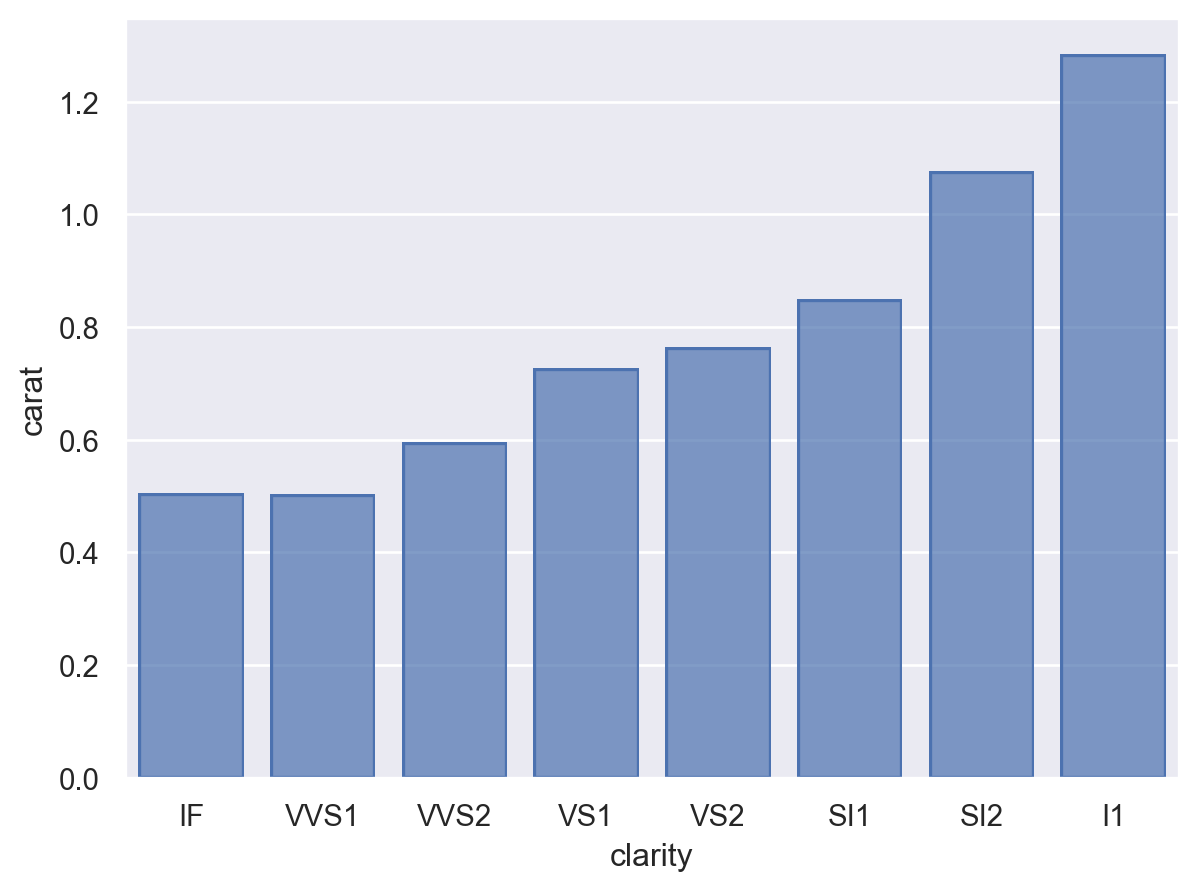Other aggregation functions can be selected by name if they are pandas methods:

```p.add(so.Bar(), so.Agg("median"))
```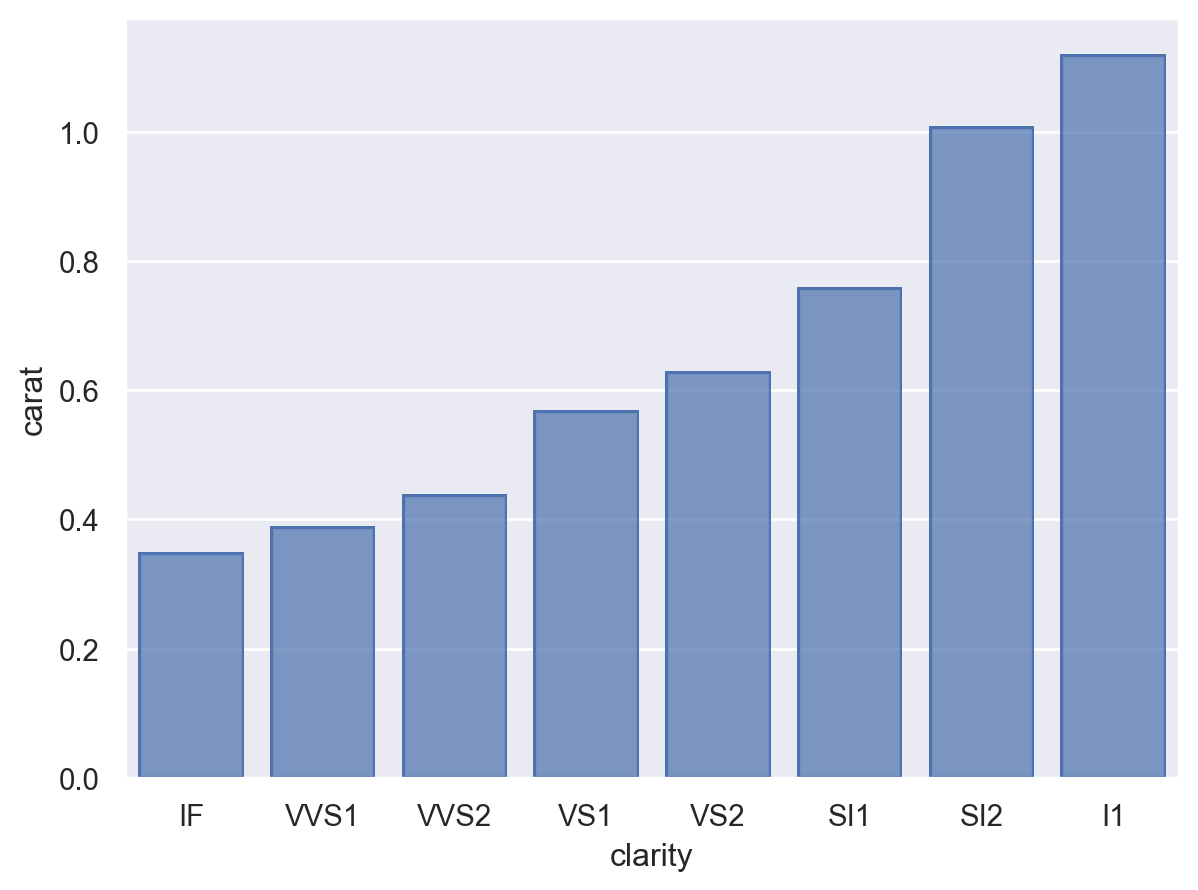It’s also possible to pass an arbitrary aggregation function:

```p.add(so.Bar(), so.Agg(lambda x: x.quantile(.75) - x.quantile(.25)))
```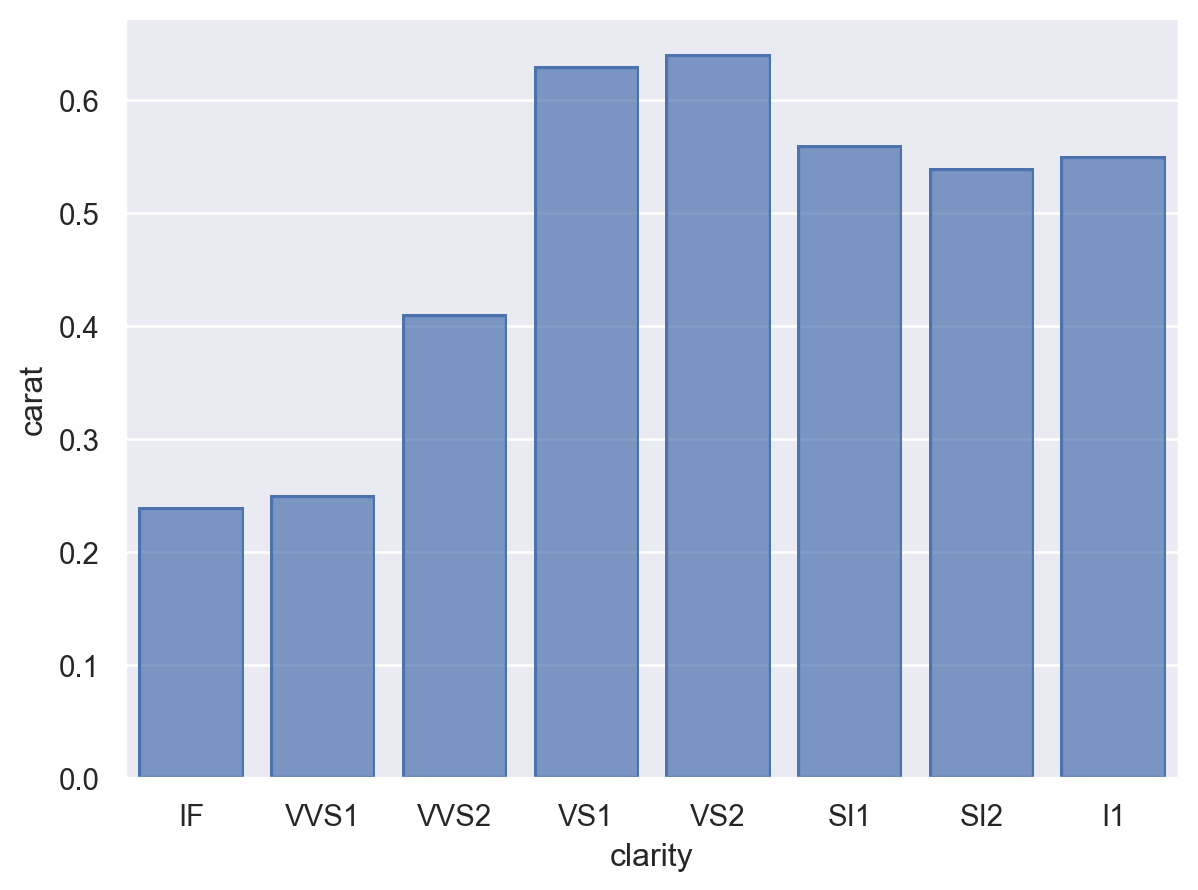When other mapping variables are assigned, they’ll be used to define aggregation groups. With some marks, it may be helpful to use additional transforms, such as `Dodge`:

```p.add(so.Bar(), so.Agg(), so.Dodge(), color="cut")
```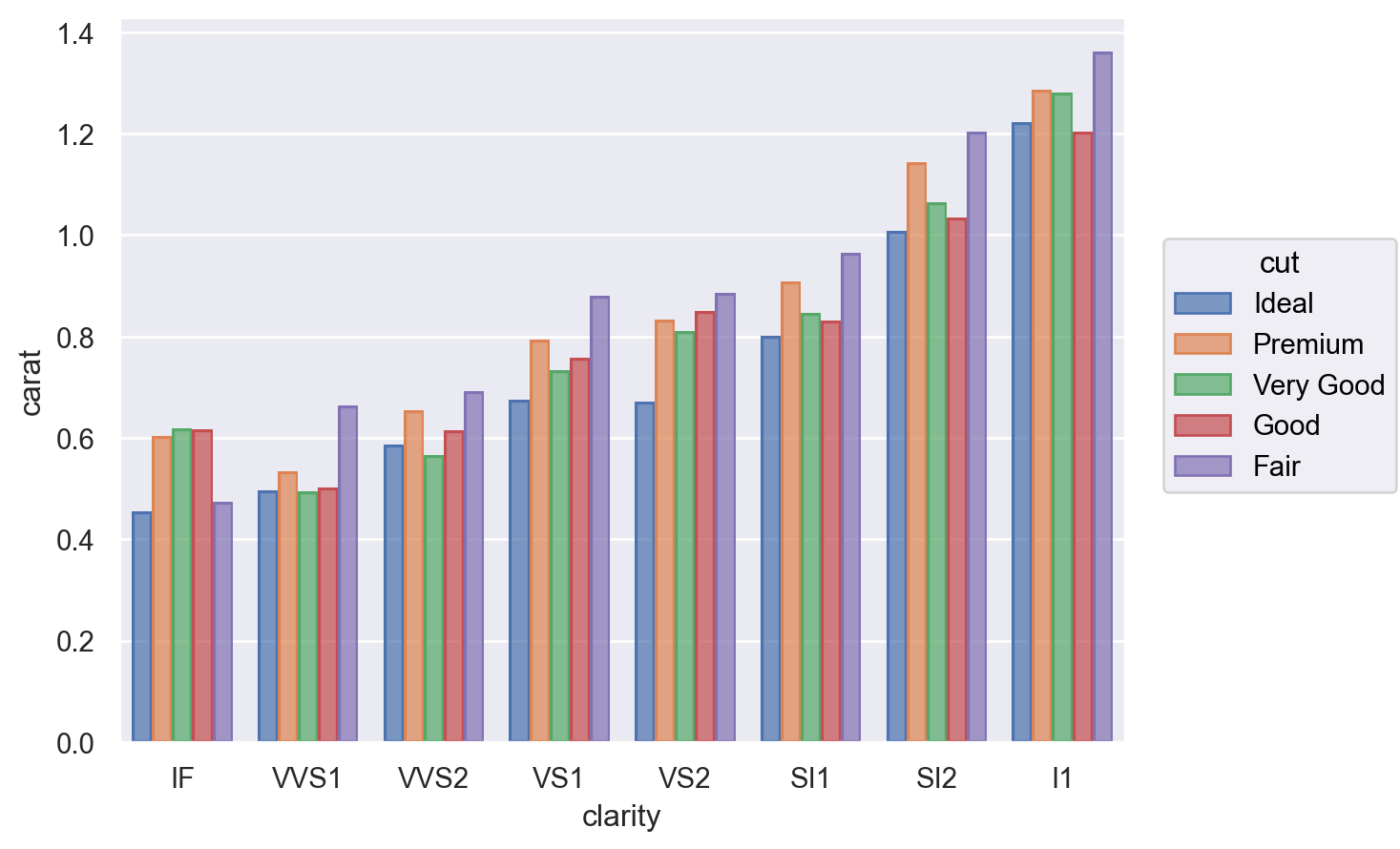The variable that gets aggregated depends on the orientation of the layer, which is usually inferred from the coordinate variable types (but may also be specified with the `orient` parameter in `Plot.add()`):

```so.Plot(diamonds, "carat", "clarity").add(so.Bar(), so.Agg())
```